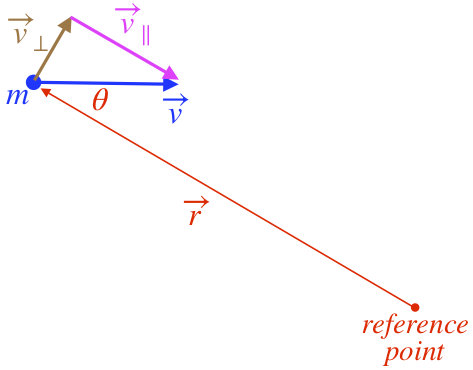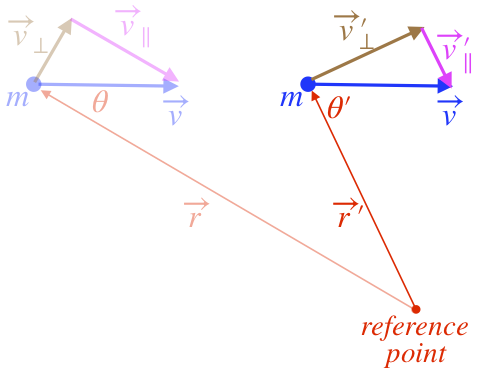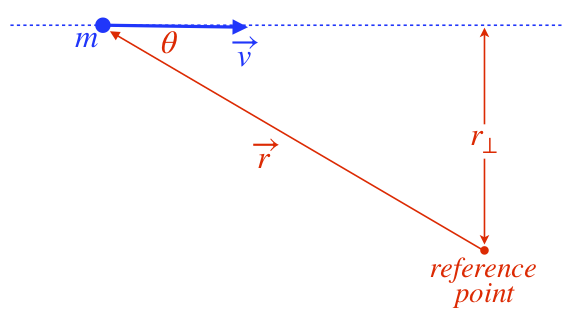$$\require{cancel}$$

# 6.1: Linking Linear and Angular Momentum

## Rotational Impulse-Momentum Theorem

By now we have a very good sense of how to develop the formalism for rotational motion in parallel with what we already know about linear motion. We turn now to momentum. Replacing the mass with rotational inertia and the linear velocity with angular velocity, we get:

$\overrightarrow p \equiv m \overrightarrow v \;\;\; \iff \;\;\; \overrightarrow L \equiv I \overrightarrow \omega$

The vector $$L$$ is called angular momentum, and it has units of:

$$\left[ L \right] = \dfrac{kg \cdot m^2}{s} = J \cdot s$$

Continuing the parallel with the linear case, the momentum is relates to the force through the impulse-momentum theorem, which is:

$\int \limits_{t_A}^{t_B} \overrightarrow F_{net} dt = \Delta \overrightarrow p_{cm} \;\;\; \iff \;\;\; \int \limits_{t_A}^{t_B} \overrightarrow \tau_{net} dt = \Delta \overrightarrow L$

While there is no need to append "cm" to the angular momentum as we do with the linear momentum, we do have to keep in mind that all of the quantities in the rotational case must be referenced to the same point. That is, the net torque requires a reference point, and the angular momentum contains a rotational inertia, which also requires a reference point.

Recall that the impulse-momentum theorem is just a repackaging of Newton's second law, and so it is with the rotational case, though there is a twist, as we will see shortly:

$\overrightarrow F_{net} = \dfrac{d\overrightarrow p_{cm} }{dt} = \dfrac{d\left(m\overrightarrow v_{cm}\right) }{dt} = m\overrightarrow a_{cm} \;\;\; \iff \;\;\; \overrightarrow \tau_{net} = \dfrac{d \overrightarrow L}{dt} = \dfrac{d\left(I\overrightarrow \omega\right) }{dt} = I\overrightarrow \alpha$

## Link Between Angular and Linear Momentum

When there are several particles in a system, we find the momentum of the system by adding the momenta of the particles:

$\overrightarrow p_{cm} = \overrightarrow {p_1 }+ \overrightarrow {p_2} + \dots$

We have a definition for the angular momentum of a rigid object, but can we define the angular momentum of a single particle, and then add up all of the angular momenta of the particles to get the angular momentum of the system, in the same way that we do it for linear momentum? The answer is yes, but we have to be careful about our reference point. That is, to add the angular momentum of every particle together to get a total angular momentum, the individual angular momenta must be measured around the same reference.

So how do we define the angular momentum of an individual particle around a certain reference point? Let's look at a picture of the situation. The particle has a mass $$m$$, a velocity $$\overrightarrow v$$, and is located at a position $$\overrightarrow r$$ with the tail of that position vector at the reference point.

Figure 6.1.1a – Angular Momentum of a Point ParticleIf this particle was a part of a rigid body rotating around the reference point, the parallel component of the velocity vector would be zero. So it makes sense to exclude that part of the velocity vector when defining the angular momentum of this particle. We know the rotational inertia of the point particle, and the relation between $$v_{\bot}$$ and $$\omega$$, so we get for the magnitude of the angular momentum:

$L_{single\;particle} = I \omega = \left[mr^2\right] \left[\dfrac{v_{\bot}}{r}\right] = mrv_{\bot} = mrv\sin\theta$

Suppose the particle continues moving free of any forces. What happens to its angular momentum? Let's look at what happens to the picture:

Figure 6.1.1b – Angular Momentum of a Point ParticleWhat a mess! The mass and velocity vector remain the same, but everything else changes. How can we determine what happens to the angular momentum? Well, have a look at Equation 6.1.2. With no force on the particle, there can't be any torque on the system, so the angular momentum must remain unchanged. It turns out there is a simpler way to look at the angular momentum, to see why this must be the case.

Figure 6.1.1c – Angular Momentum of a Point ParticleWe can define the quantity $$r_{bot}$$ in a manner similar to how we defined moment arm – it is the perpendicular distance from the reference point to the line along which the particle is moving. Doing this gives us an alternative way of writing the magnitude of the particle's angular momentum. Using the fact that $$r_{\bot}=r\sin\theta$$, we have:

$L_{single\;particle} = mrv_{\bot} = mrv\sin\theta = mvr_{\bot}$

Now it is quite easy to see that the angular momentum of the particle doesn't change while it moves – it keeps the same mass and speed, and stays on the same line, so $$r_{\bot}$$ doesn't change either.

Angular momentum is a vector, so what direction does it have here? Going back to the idea of this particle being part of a rigid object, it's clear that this object would be rotating clockwise around the reference, so from the right hand rule, the vector must point into the page. We would like a mathematical expression of this, and as with the case of torque, it comes from the cross product. The two vectors involved are the position vector and the velocity vector, and indeed we see that the following cross product results in the correct direction, and takes care of the $$\sin\theta$$ contribution as well:

$\overrightarrow L_{single\;particle} = m\overrightarrow r \times \overrightarrow v = \overrightarrow r \times \overrightarrow p$

This is a nice, compact expression of the relation between the linear momentum of a particle and its angular momentum around a reference point. To see this relation come full circle, imagine that a force is exerted on the particle. This would cause the momentum to change. It would also result in a torque on the system about the reference point, causing the angular momentum to change. Taking the derivative of Equation 6.1.7 with respect to time gives:

$\overrightarrow \tau = \dfrac{d\overrightarrow L}{dt} = \dfrac{d}{dt}\left(\overrightarrow r \times \overrightarrow p\right) = \dfrac{d\overrightarrow r}{dt} \times \overrightarrow p + \overrightarrow r \times \dfrac{d\overrightarrow p}{dt} = \overrightarrow v \times \overrightarrow p + \overrightarrow r \times \overrightarrow F$

The velocity vector is parallel to the momentum vector, so the cross product in the first term is zero, leaving us with a relation between torque and force that we have seen before (Equation 5.5.6).

Now that we can deal with the angular momentum of a single particle relative to some reference point, we can simply add the contributions of many such particles within a system, relative to the same reference point:

$\overrightarrow L_{system} = \overrightarrow r_1 \times \overrightarrow p_1 + \overrightarrow r_2 \times \overrightarrow p_2 + \dots$

Note that these particles may be part of a rigid object, or may not be bound to each other at all. If they happen to be bound into a single rigid object rotating around a fixed point on the object, then the result is more easily expressed in terms of the rigid object's rotational inertia and angular velocity (Equation 6.1.1):

$\begin{array}{l} \overrightarrow L_{rigid\;object} && = && \overrightarrow r_1 \times m_1\overrightarrow v_1 + \overrightarrow r_2 \times m_2\overrightarrow v_2 + \dots \\ && = && \left[r_1\widehat r_1\right] \times m_1\left[v_1\widehat v_1\right] + \left[r_2\widehat r_2\right] \times m_2\left[v_2\widehat v_2\right] + \dots \\ && = && \left[r_1\widehat r_1\right] \times m_1\left[r_1\omega\widehat v_1\right] + \left[r_2\widehat r_2\right] \times m_2\left[r_2\omega\widehat v_2\right] + \dots \\ && = && m_1 r_1^2\left[\omega\widehat r_1 \times \widehat v_1\right] + m_2 r_2^2\left[\omega\widehat r_2 \times \widehat v_2\right] + \dots \\ && = && m_1 r_1^2\left[\omega\widehat \omega\right] + m_2 r_2^2\left[\omega\widehat \omega\right] + \dots \\ && = && I\overrightarrow\omega \end{array}$

Consider next an extended object that is not rotating, but is moving in a straight line relative to some reference point. Despite the fact that it is not rotating, it can have angular momentum relative to that reference point. Writing the angular momentum of the whole object as a sum of the angular momenta of its particles, we get:

$\overrightarrow L_{not-rotating\:extended\:object} = m_1\overrightarrow r_1 \times \overrightarrow v_1 + m_2\overrightarrow r_2 \times \overrightarrow v_2 + \dots$

With the object not rotating and all the particles held rigidly in place, every particle has the same velocity, which equals the velocity of the object's center of mass, so this can be factored out of all the cross products, giving:

$\overrightarrow L_{not-rotating\:extended\:object} = \left(m_1\overrightarrow r_1 + m_2\overrightarrow r_2 + \dots\right) \times \overrightarrow v_{cm} = \left(\dfrac{m_1\overrightarrow r_1 + m_2\overrightarrow r_2 + \dots}{M}\right) \times \left(M\overrightarrow v_{cm}\right) = \overrightarrow r_{cm} \times \overrightarrow p_{cm}$

What this means is that an extended object moving in a straight line has the same angular momentum relative to a reference point as a point particle located at the object's center of mass, with the same mass and velocity.

If the extended object has both its center of mass moving at a constant velocity relative to the reference point and it is also rotating around an axis through its own center of mass, then things get complicated. We won't into the details of the most general case, but it is not unreasonable to consider the case of the linear velocity lying in the plane perpendicular to the rotation vector (e.g. an object moving within this screen while rotating around an axis perpendicular to this screen – see Figure 6.1.2).

Figure 6.1.2 – Total Angular MomentumThe total angular momentum comes out to be reminiscent of the parallel-axis theorem and of the kinetic energy being the sum of linear and rotational parts:

$\overrightarrow L_{tot} = \overrightarrow L_{rotation\; around \:cm} + \overrightarrow L_{cm \; moving \; by\;reference \; point} = I_{cm} \overrightarrow \omega + \overrightarrow r_{cm} \times \overrightarrow p_{cm}$

An interesting and important consequence of this is that an object that is only rotating around its center of mass (but not moving linearly) has the same angular momentum measured relative to every reference point.

Example $$\PageIndex{1}$$

The center of a uniform solid disk is attached to a thin uniform rod of equal mass at its end. The radius of the disk is one-third the length of the rod. The rod is held fixed and is free to pivot about at its other end. A constant magnitude of force is exerted tangent to the edge of the disk at the point farthest from the pivot for a short time. The is no gravity present.The system starts from rest, and after the short time, it has some angular momentum relative to the pivot, with the disk and rod each contributing a portion of it. Find the ratio of the angular momenta contributed by each part if...

1. ... the disk is held rigidly to the rod (i.e. it is not free to rotate around its center).
2. ... the disk can rotate freely about its center.
Solution

a. Even though the disk is held rigidly at it center by the rod, it still rotates relative to its center (if you don't see this, imagine painting an arrow on the side of the disk, and focus only on the disk as the whole object spins – clearly the orientation of the disk rotates). In fact, the disk rotates around its center at precisely the same rate as the whole object rotates (the aforementioned painted arrow takes the same amount of time to come all the way around as the object itself). We can compute the angular momentum contribution of the disk and rod in terms of this common angular speed. For the rod it is easy:

$L_{rod} = I_{rod}\omega = \frac{1}{3}ml^2\omega \nonumber$

The disk requires a bit more effort, since its angular momentum consists of a part around its center of mass and a part from the linear motion going around the pivot:

$L_{disk} = I_{disk}\omega = \frac{1}{2}mR^2\omega + mr_\bot v= \frac{1}{2}m\left(\frac{1}{3}l\right)^2 + ml\left(l\omega\right)\omega = \frac{19}{18}ml^2\omega \nonumber$

The ratio of the angular momenta is:

$\dfrac{L_{rod}}{L_{disk}} = \boxed{\frac{6}{19}} \nonumber$

b. This is significantly more difficult than part (a), because the angular velocity of the disk is not equal to that of the rod. Hopefully it is clear that the rod must rotate. That is, just because the disk is free to rotate, it doesn't mean that the force will not affect the rod's motion. To see this, consider a FBD of the whole system. If $$F$$ is the only force, then the disk's center of mass must be accelerating, which it is not doing if it is only spinning in place. If there is a balancing force to keep the disk's center of mass in place, then it can only come from the point of contact with the rod. But then the equal-and-opposite force on the end of the rod by the disk will make the rod rotate.

Okay, so we need the separate angular momenta (about the pivot) of the disk and rod to determine the ratio. The angular momentum of the disk is once again the sum of its angular momentum about its center and that which comes from its linear motion around the pivot (which is directly related to the rotational speed of the rod). Calling the rotational speed of the rod $$\omega_1$$, and the rotational speed of the disk around its center $$\omega_2$$, we have the following angular momenta for the two pieces:

$\begin{array}{l} L_{rod} = \frac{1}{3}ml^2\omega_1 \\ L_{disk} = \frac{1}{2}mR^2\omega_2 + ml^2\omega_1 = ml^2\left(\frac{1}{18}\omega_2 + \omega_1\right)\end{array} \nonumber$

Note that so far, this same analysis applies to part (a), with the difference being that in part (a) the disk is turning at the same rate as the rod: $$\omega_2=\omega_1=\omega$$. What we need for this case is a way to relate $$\omega_1$$ and $$\omega_2$$, which are no longer equal. Both come after a torque is applied for an equal time, but the net torque applied to the rod about the pivot (which determines $$\omega_1$$) is different from the net torque applied to the disk about its center (which determines $$\omega_2$$). To get a handle on this, a pair of FBDs are helpful:A quick explanation of these FBDs: $$N$$ is the normal force by the axle on the disk, reacting to the applied force, as discussed in the first paragraph above. $$T$$ is the "tension" force keeping the disk moving in a circle. $$V$$ is the vertical force by the pivot that makes sure there is a net force which keeps the center of mass of the rod moving in a circle. Neither $$T$$ nor $$V$$ play a role in either torque. We'll say that $$F$$ and $$N$$ act for a time $$\Delta t$$.

The net torque on the rod about the pivot is $$Nl$$. Multiply this by the time it acts (and remembering that it starts from rest), we have, from the impulse-momentum theorem:

$Nl\Delta t = I_{rod\;about\;pivot}\; \omega_1 = \frac{1}{3}ml^2 \omega_1 \;\;\; \Rightarrow \;\;\; N\Delta t = \frac{1}{3}ml \omega_1\nonumber$
The net torque on the disk about its center is $$FR$$, so:

$FR\Delta t = I_{disk\;about\;center}\; \omega_2 = \frac{1}{2}mR^2 \omega_2 \;\;\; \Rightarrow \;\;\; F\Delta t = \frac{1}{6}ml \omega_2 \nonumber$
[Note: While it might appear as though this rotational impulse determines the rotational motion of the disk relative to the rod, it does not. The resulting motion is the total angular velocity (relative to the lab). If this force was zero, and the rod is made to turn without any torque on the disk (i.e. the force is applied to the rod instead of the disk), the disk would maintain its orientation relative to the lab as the rod rotates, turning relative to the rod in the opposite direction at the same rate that the rod rotates. In this case, $$\omega_2$$ would be zero, which matches the zero value of $$F$$.]

We need one more equation, and it comes from the linear impulse-momentum theorem for the disk. From the FBD, we see that the net force on the disk is $$F-N$$, and this results in a change of (tangential) momentum of $$mv_{cm}$$. The final linear velocity of the disk's center of mass is directly related to $$\omega_1$$ (it moves with the end of the rod) so:

$\left(F-N\right)\Delta t = mv_{cm} = ml\omega_1 \nonumber$
Putting these last three equations together gives a relationship between $$\omega_1$$ and $$\omega_2$$:

$\frac{1}{6}ml \omega_2 - \frac{1}{3}ml \omega_1 = ml\omega_1 \;\;\; \Rightarrow \;\;\; \omega_2 = 8\omega_1 \nonumber$

Finally, we put this into the angular momentum for the disk that we found earlier, and compute the ratio:

$L_{disk} = ml^2\left(\frac{1}{18}\left[ 8\omega_1\right] + \omega_1\right) = \frac{13}{9}ml^2 \omega_1 \;\;\; \Rightarrow \;\;\; \dfrac{L_{rod}}{L_{disk}} = \boxed{\frac{3}{13}} \nonumber$

It makes sense that more of the angular momentum goes into the disk in the case where it spins faster than the rod. If you really want to go down the rabbit hole, you can explore a comparison of the kinetic energy given to the system in the two cases. As we have seen before, just because the system has the same angular momentum in both of these cases (same torque on the system for the same period of time), it doesn't mean that they have the same kinetic energy when there are two components of the system that can distribute the momentum differently between them.